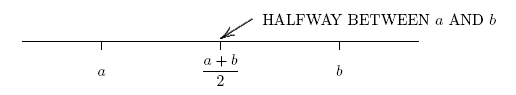﻿ Average of Two Signed Numbers
AVERAGE OF TWO SIGNED NUMBERS
by Dr. Carol JVF Burns (website creator)
Follow along with the highlighted text while you listen!
• PRACTICE (online exercises and printable worksheets) …
• ... and lots MORE PRACTICE!
Want more details, more exercises?

A teacher reports an average grade on a test.
What do these figures mean?
The purpose of this section is to discuss the concept, the computation, and some important properties of averaging.

To average two numbers means to add the numbers together, and then divide by $\,2\,$. Thus:

$$\text{the average of } \,a\, \text{ and } \,b\, \text{ is } \,\frac{a+b}{2}$$

Averaging two different numbers always gives the number exactly halfway between, as illustrated below:In this web exercise, you will compute averages of two numbers, where the numbers can be $\,-10, -9, \ldots, 9, 10\,$.
You should be able to do this exercise without a calculator!
It is good practice with mental arithmetic, and will reinforce your skills with addition of signed numbers.

There are two key ideas to keep in mind:

• If the two numbers being averaged are close to each other,
just visualize the number line and picture the number that is exactly halfway between.
• If the numbers being averaged are far enough apart that you can't easily decide which number is halfway between,
then do the arithmetic:
add the two numbers and divide by $\,2\,$.

Clearly, the formula $\,\frac{a+b}2\,$ gives some number;
but how do we know that the number given by this formula is really, always, halfway between $\,a\,$ and $\,b\,$?
Although repeated trials (with lots of different numbers) is pretty convincing, it is of course impossible to check every pair of real numbers.
To see a proof, read the text!

EXAMPLES:
Question: What is the average of $\,-3\,$ and $\,-5\,$?
Solution: Visualize $\,-3\,$ and $\,-5\,$ on the number line.
What number is halfway between?
Answer:   $-4$
Question: What is the average of $\,7\,$ and $\,10\,$?
Solution: Visualize $\,7\,$ and $\,10\,$ on the number line.
What number is halfway between?
Answer:   $8.5$
Question: What is the average of $\,-7\,$ and $\,9\,$?
Solution: These numbers are too far apart to easily visualize halfway between.
Do the arithmetic:   $\frac{-7+9}2 = \frac{2}2 = 1$
Answer:   $1$
Master the ideas from this section

When you're done practicing, move on to either:
Average of Signed Numbers

OR

a beautiful application of averaging concepts

In this exercise, you must write your answers in decimal form (as needed).
That is, write (say) $\,2.5\,$, not $\,5/2\,$.